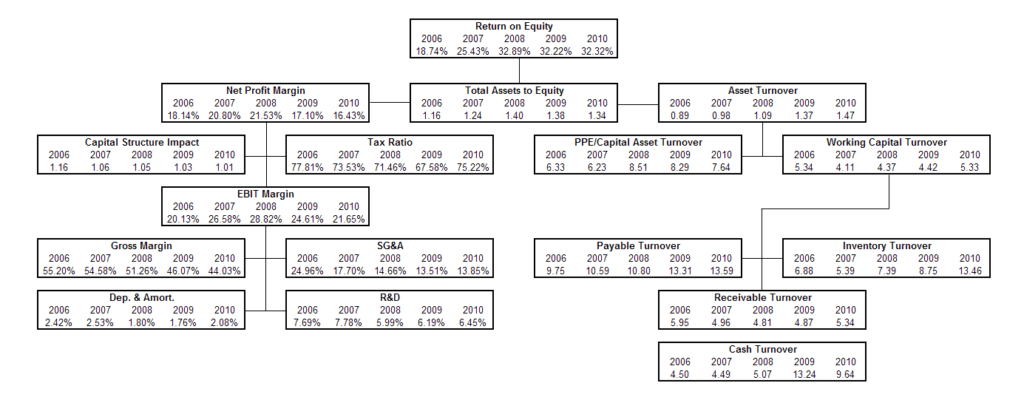# Financial Ratios Cheat Sheet

## Financial Ratios Cheat Sheet

CFI’s Financial Ratios cheat sheet is a pdf ebook, available for anyone to download for free. The cheat sheet goes over the essential financial ratios that a Financial Analyst uses to analyze a business. Below is an image of the Pyramid of Ratios, a common tool used by industry professionals to analyze a company’s efficiency, profitability, and solvency and to show how they are interconnected.

### Pyramid of RatiosImage: Pyramid of Ratios from CFI’s Financial Analysis Course

### Financial Ratios eBook

In this e-book you will find the following Financial Ratios:

### Liquidity Ratios

• Current Ratio / Working Capital Ratio
• Quick Ratio / Acid Test Ratio
• Cash Ratio
• Times Interest Earned
• Capex to Operating Cash Ratio
• Defensive Interval Ratio
• Operating Cash Flow Ratio
• Times Interest Earned (Cash Basis) Ratio

### Efficiency Ratios

• Accounts Receivable Turnover
• Accounts Receivable Days
• Asset Turnover Ratio
• Inventory Turnover Ratio
• Inventory Turnover Days (Day Sales in Inventory)
• Capex to Operating Cash Ratio

### Leverage Ratios

• Debt to Equity Ratio
• Equity Ratio
• Debt Ratio

### Financial Ratios Cheat Sheet – Profitability Ratios

• Gross Profit Margin Ratio
• Net Profit Margin
• Return on Assets (ROA)
• Return on Capital Employed (ROCE)
• Return on Equity (ROE)
• Operating Profit Margin

### Multiples Valuation Ratios

• Price Earnings Ratio (P/E)
• EV/EBITDA Ratio
• EV/EBIT Ratio
• EV/Revenue Ratio

### Financial Ratios Cheat Sheet – Use of Ratios in Financial Analysis

There are various types of financial analysis, and the use of ratios is an indispensable aspect of all of them. This cheat sheet is a concise and organized source of information on the essential ratios for financial analysis. It can be used as a quick reference to the information. Ratios are a powerful analytical tool for performing both cross-sectional and time-series analysis, as ratios can be compared across time periods and industries. It is also important, however, to be aware of the limitations of ratio analysis.